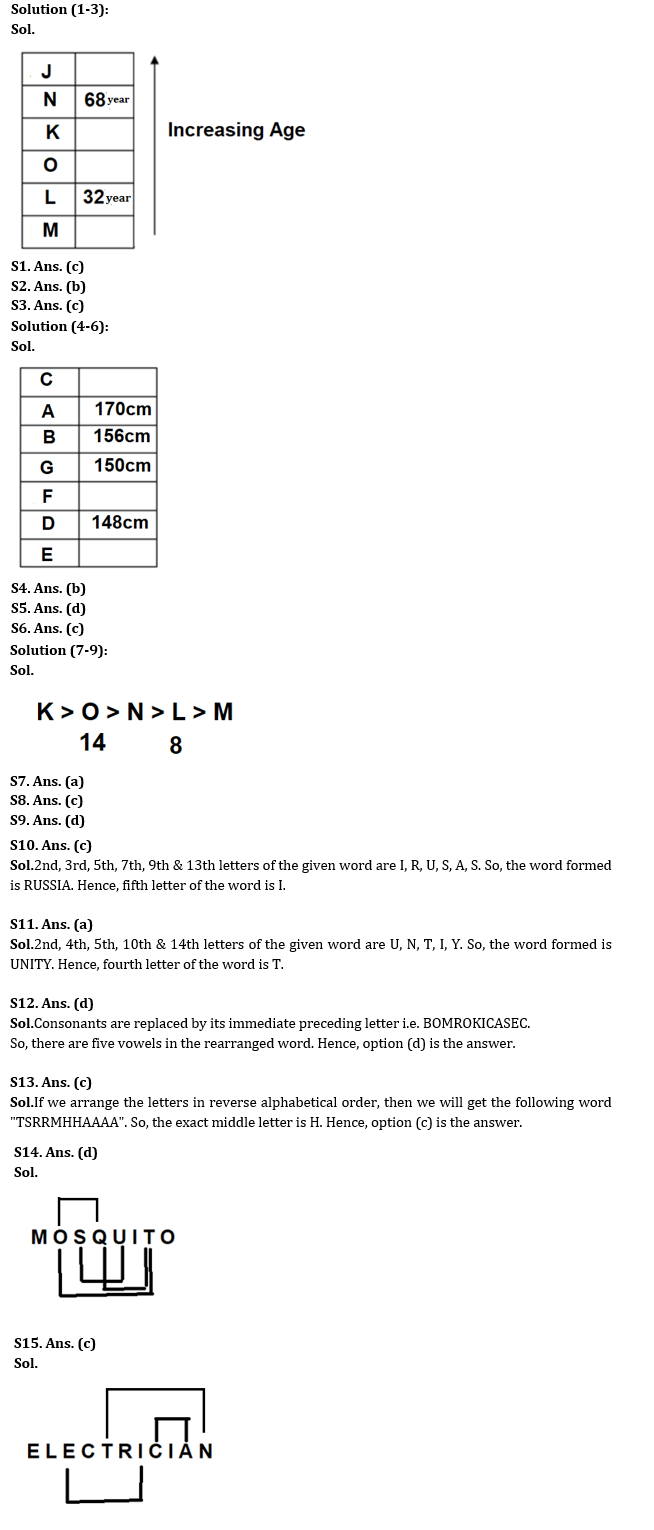Latest Banking jobs   »   Reasoning Ability Quiz For IBPS RRB...

# Reasoning Ability Quiz For IBPS RRB PO Prelims 2022- 17th June

Directions (1-3): Study the information carefully and answer the questions accordingly.
There are six people J, K, L, M, N, and O having different ages but not necessarily in the same order. K is older to more than two people. Only one person is older than N. K is older than L, who is not the youngest. The age of O is more than 32year. The one, who is the second youngest, is 32year old and the one, who is second oldest, is 68year old. J is older than N and O.

Reasoning Quiz For IBPS RRB PO Prelims 2022 17th June CHECK IN HINDI

Q1. Who is the youngest?
(a) K
(b) J
(c) M
(d) Either k or M
(e) None of these

Q2. If the sum of the age of K and L is 74 years than what could be the age of O (in year)?
(a) 43
(b) 40
(c) 44
(d) 30
(e) 31

Q3. If the sum of the age of K and N is 112 years than what will be the age of N(in year)?
(a) 43
(b) 46
(c) 44
(d) 57
(e) None of these

Directions (4-6): Study the information carefully and answer the questions accordingly.
Seven people A, B, C, D, E, F, and G are of different heights but not necessarily in the same order. A is taller than E, but shorter than C. The height of G, who is taller than D, is 150cm. The one, who is second shortest, is 148cm in height. Two people are shorter than F. Neither A nor E has the height, which is a multiple of 3. One of them has a height of 156cm. C is taller than G and F and does not have a height of 170cm. The one, who is second tallest, is 170cm in height. D is not the shortest among all.

Q4. Who is the tallest in the group?
(a) B
(b) C
(d) D
(d) A
(e) None of these

Q5. How many people are shorter than G?
(a) Two
(b) One
(c) Four
(d) Three
(e) None of these

Q6. What could be the possible height of E?
(a) 159cm
(b) 155cm
(c) 140cm
(d) 149cm
(e) 150 cm

Directions (7-9): Study the information carefully and answer the questions accordingly.
Five students i.e., M, N, O, K, and L study in class X. All of them have different height. L is shorter than N and taller than M. Not more than one student is taller than K. N is shorter than O. O’s height is 14ft and L’s height is 8ft. O is shorter than K.

Q7. Which of the following is the possible height of K (in feet)?
(a) 15
(b) 12
(c) 8
(d) 10
(e) 9

Q8. How many students are taller than L?
(a) One
(b) Two
(c) Three
(d) Four
(e) None of the above

Q9. Which of the following statement is true?
(a) O is shortest.
(b) N’s height is 16 feet
(c) M is taller than O
(d) L is shorter than O
(e) None of the above

Q10. If it is possible to make a six-letter meaningful word from 2nd, 3rd, 5th, 7th, 9th & 13th letters from the left end of the word “CIRCUMSTANCES”, then which will be the 5th letter of the meaningful word? If more than one such word can be formed then ‘X’ is your answer. If no such word is formed, then ‘Y’ is your answer.
(a) Y
(b) A
(c) I
(d) S
(e) X

Q11. If it is possible to make a five-letter meaningful word from 2nd, 4th, 5th, 10th & 14th letters from the left end of the word “QUANTITATIVELY”, then which will be the 4th letter of the meaningful word? If more than one such word can be formed then ‘J’ is your answer. If no such word is formed, then ‘Y’ is your answer.
(a) T
(b) Y
(c) I
(d) N
(e) J

Q12. If all the consonants of the Word “CONSOLIDATED” are replaced by immediately preceding letter in the alphabetical series, how many vowels are there in the rearranged word?
(a) Two
(b) Three
(c) Four
(d) Five
(e) None of the above

Q13. Which of the following letter will be the exact middle letter if the letters of the word “MAHARASHTRA” are arranged in Reverse alphabetical order from left to right?
(a) M
(b) R
(c) H
(d) S
(e) None of the above

Q14. How many pairs of letters are there in the word “MOSQUITO” which has as many letters between them in the word as in the alphabetical series (both in forward and backward direction)?
(a) One
(b) Two
(c) Three
(d) Four
(e) None of the above

Q15. How many pairs of letters are there in the word “ELECTRICIAN” which has as many letters between them in the word as in the alphabetical series (both in forward and backward direction)?
(a) One
(b) Two
(c) Three
(d) Four
(e) None of the above

Solutions#### Congratulations!